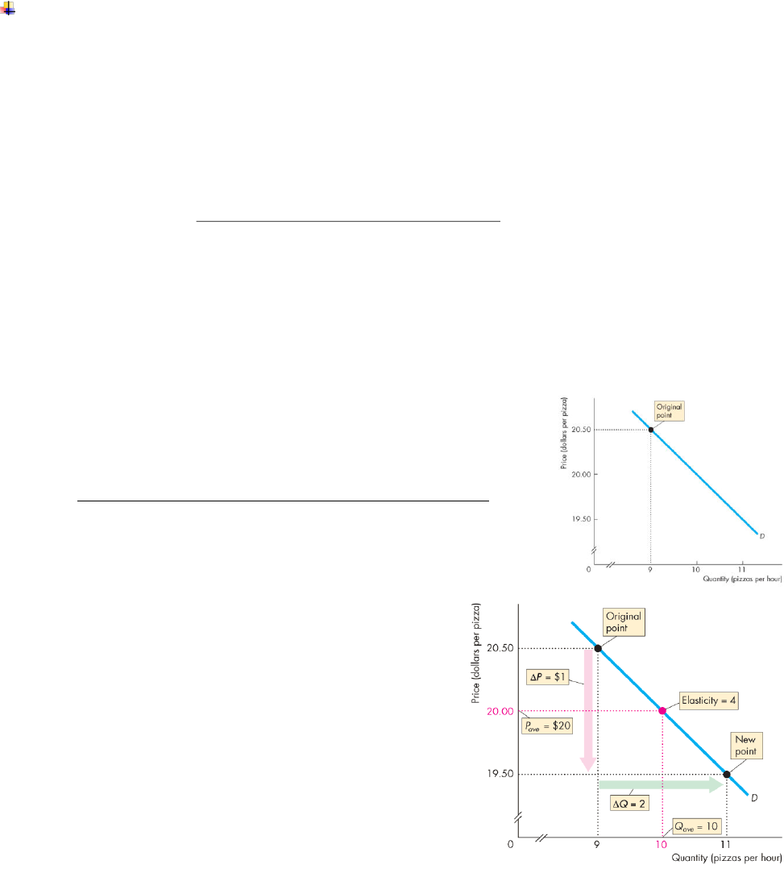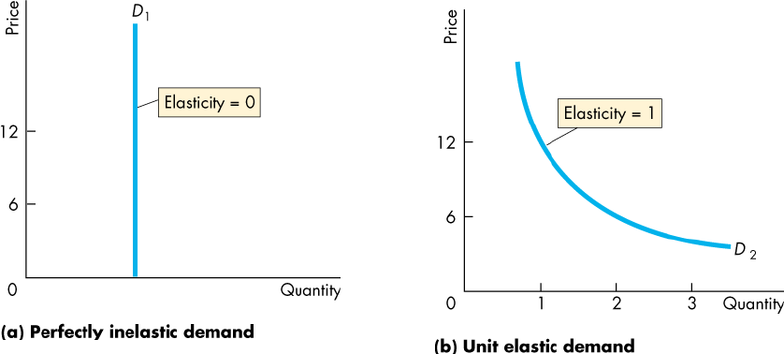Class Notes (1,100,000)
CA (650,000)
UW (20,000)
ECON (1,000)
ECON101 (500)
Lecture 7

# ECON101 Lecture Notes - Lecture 7: Demand Curve

Department
Economics
Course Code
ECON101
Professor
Predrag Rajsic
Lecture
7

This preview shows page 1. to view the full 5 pages of the document.ECON 101 Lec 7 Price Elasticity of Demand
Price Elasticity of Demand
- To measure responsiveness we need a measure that is independent of units of
measurement.
- Elasticity is such a measure.
- price elasticity of demand - a units-free measure of the responsiveness of the
quantity demanded of a good to a change in its price when all other influences on
Calculating price elasticity of demand
Formula:
To calculate the price elasticity of demand:
- We express the change in price as a percentage of the average pricethe average
of the initial and new price, …
- and we express the change in the quantity demanded as a percentage of the
average quantity demandedthe average of the initial and new quantity.
Figure calculates the price elasticity of demand for pizza.
- Initially, the price of a pizza is \$20.50 and the
quantity demanded is 9 pizzas an hour.
- The price of a pizza falls to \$19.50 and the quantity
demanded increases to 11 pizzas an hour.
- The price falls by \$1 and the quantity demanded
increases by 2 pizzas an hour.
- The average price is \$20 and the
average quantity demanded is 10 pizzas
an hour.
- The percentage change in quantity
demanded, %ΔQ, is calculated as …
ΔQ/Qave × 100, which is
(2/10) × 100 = 20%.
- The percentage change in price, %ΔP, is
calculated as ΔP/Pave × 100, which is
(\$1/\$20) × 100 = 5%.
- The price elasticity of demand is
%ΔQ/%ΔP = 20%/5%
= 4
Average Price and Quantity
- By using the average price and average quantity, we get the same elasticity value
regardless of whether the price rises or falls.
Percentage change in the quantity demanded
Percentage change in the price

Unlock to view full version

Only page 1 are available for preview. Some parts have been intentionally blurred.o Percentages and Proportions
- The ratio of two proportionate changes is the same as the ratio of two percentage
changes.
- %ΔQ/%ΔP = ΔQ/ΔP
A Units-Free Measure
- Elasticity is a ratio of percentages, so a change in the units of measurement of price
or quantity leaves the elasticity value the same.
Minus Sign and Elasticity
- The formula yields a negative value, because price and quantity move in opposite
directions.
- But it is the magnitude, or absolute value, that reveals how responsive the quantity
change has been to a price change.
Inelastic and Elastic Demand
- Demand can be inelastic, unit elastic, or elastic, and can range from zero to infinity.
- If the quantity demanded doesn’t change when the price changes, the price
elasticity of demand is zero and the good has a perfectly inelastic demand.
1) Figure (a) illustrates the case of a good that has a perfectly inelastic demand.
- The demand curve is vertical.
2) Figure (b) illustrates this casea demand curve with ever declining slope.
- If the percentage change in the quantity demanded equals the percentage change
in price, the price elasticity of demand equals 1 and the good has unit elastic
demand.
· Note: